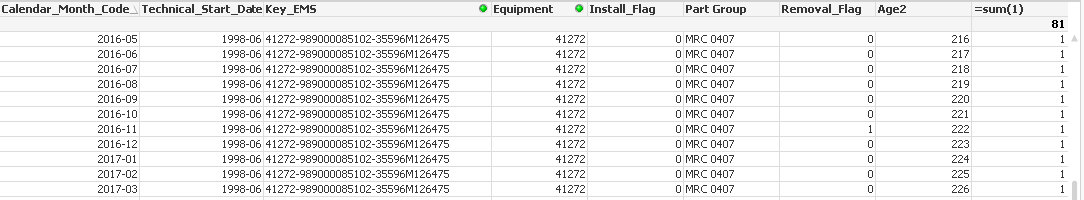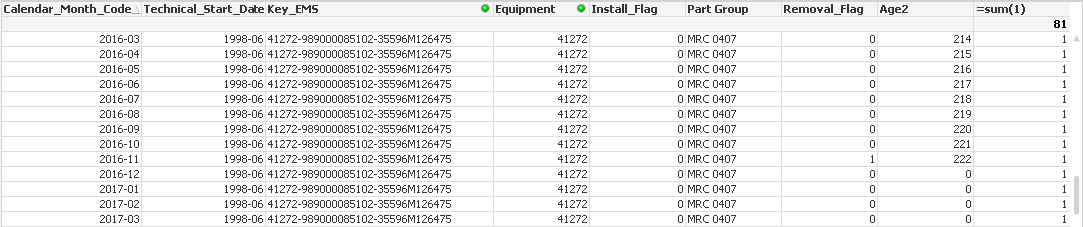# QlikView App Development

Discussion Board for collaboration related to QlikView App Development.

Announcements
Make your voice heard! Participate in the 2020 Wisdom of Crowds® Survey. BEGIN SURVEY
Highlighted
Contributor III

## Nested if help in edit script

Hi All,

I have the below if else condition written in edit script

if(Previous(Key_EMS) <> Key_EMS,(\$(MonthDiff(Technical_Start_Date, Calendar_Month_Code))),

if(Previous(Key_EMS) = Key_EMS,(\$(MonthDiff(Technical_Start_Date, Calendar_Month_Code))),

if(Previous(Install_Flag) = 1 and Previous(Removal_Flag) = 1, 0,

if(Previous(Install_Flag) = 1 and Previous(Removal_Flag) = 0, rangesum(peek('Age'),1),

if(Previous(Install_Flag) = 0 and Previous(Removal_Flag) = 1, 0,

if(Previous(Peek('Age2')) = 0, 0, Rangesum(Peek('Age2'),1))))))) as Age2

The first 2 if conditions are working fine but from the third line it is not working.

Thanks,

Bharat

2 Replies
HighlightedMVP

## Re: Nested if help in edit script

There are at least two things that doesn't make much sense in the logic:

1. The first condition and the second condition will always be satisfied since they are opposite conditions. One of the two will always be true so none of the conditions after the two will ever be tested at all.
2. What will be calculated by any of the first two conditions will be exactly the same since the expression after the condition is the same for both.

My guess without knowing what you want to calculate here is that the second line overstriked in red should be removed completely.

if(Previous(Key_EMS) <> Key_EMS,(\$(MonthDiff(Technical_Start_Date, Calendar_Month_Code))),

if(Previous(Key_EMS) = Key_EMS,(\$(MonthDiff(Technical_Start_Date, Calendar_Month_Code))),

if(Previous(Install_Flag) = 1 and Previous(Removal_Flag) = 1, 0,

if(Previous(Install_Flag) = 1 and Previous(Removal_Flag) = 0, rangesum(peek('Age'),1),

if(Previous(Install_Flag) = 0 and Previous(Removal_Flag) = 1, 0,

if(Previous(Peek('Age2')) = 0, 0, Rangesum(Peek('Age2'),1)))))) ) as Age2

Note that you will also have to remove a paranthesis at the end before the "as Age2".

Highlighted
Contributor III

## Re: Nested if help in edit script

Hi Petter,

Thank you so much for your reply. i guess the order by is making an issue.if am giving the below order by

Calendar_Month_Code,Key_EMS,Equipment,[Part Group] ;

as per the condition(except for KEY EMS missing all the conditions are going wrong) written whenever removal flag is there instead of getting 0 i am getting continuous number: Image for your reference:But when i change the order by i am getting write numbers for all the conditions except for Key ems missing.

order by Equipment,[Part Group],  Key_EMS,Calendar_Month_Code; Below image for reference:I am attaching the file for reference.